## Nuclear Notation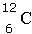Looking at the symbol above, you can identify many things about this element. The element symbol is given. To the left of the symbol, the top number is the mass number, which is also given the symbol A. Remember that the mass number = protons + neutrons. The bottom number to the left of the symbol is the atomic number, which is also given the shorthand symbol of Z. Remember that the atomic number = protons, and also the charge of the nucleus. The mass number is equal to the number or nucleons (particles in the nucleus).

Looking at the symbol above, the element is carbon. It has 6 protons and 6 neutrons (12 – 6 = 6).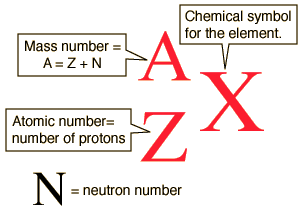The atomic number (Z) for an element does not change, but the mass number (A) can change, as neutrons are added or removed from the nucleus. Look at the nuclear notation below for an isotope of carbon.

A = 14

X = 6

The atomic number is 6. This atom of carbon has 6 protons (remember, all carbon atoms have 6 protons).

The mass number is 14. This atom of carbon has 8 neutrons (14 – 6 = 8).

Sometimes, all of this information is not necessary, and a simpler method for writing symbols is needed. Hyphen notation is a shortened version of the nuclear notation. In hyphen notation, the first element above will look like this: C-12

The element symbol is given, then a hyphen, then the mass number (A). As the atomic number never changes, and can be readily obtained from any periodic table, the hyphen notation is commonly preferred. What is the hyphen notation for the second symbol above? (Highlight to reveal answer:  C-14)

## Alpha Decay

Alpha decay usually only occurs in elements with atomic numbers of 83 and above. Alpha decay always has a product that includes a helium-4 nucleus. This helium-4 nucleus is what is known as the alpha particle or α-particle.This chemical equation represents a Uranium-238 atom undergoing alpha decay. Notice the α-particle is one product.

To determine the other product, subtract the mass number of He from the mass number of the U.

238 – 4 = 234 is the mass number of the other product.

Now, subtract the atomic number of He from the atomic number of U.

92 – 2 = 90 is the atomic number of the other product.

To determine the element symbol, look up atomic number 90 on the periodic table.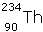is the symbol for the element with a mass number of 234 and an atomic number of 90.Uranium-238 is called the parent nuclide and thorium-234 is called the daughter nuclide.

Try another one.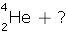To determine the mass number of the product, subtract 263 – 4 = 259.

To determine the atomic number of the product, subtract 106 – 2 = 104.

To determine the symbol of the product, look up on the periodic table atomic number 104.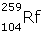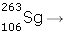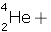What is the parent nuclide? (Highlight to reveal answer: seaborgium-263 )

What is the daughter nuclide? (Highlight to reveal answer: rutherfordium-259 )

## Beta Decay

Beta decay occurs when a negatively charged electron is emitted from the nucleus. Remember that the mass of an electron is negligible when compared to the mass of an atomic nucleus. Because of this, the mass of the nucleus that undergoes beta decay is only decreased a tiny amount, an amount so small that it can be ignored. The mass number in beta decay does not change. Remember also that the nucleus only contains protons and neutrons. Where does the electron that is emitted come from? A neutron is changed into a proton. When this occurs, an electron is formed. That electron is emitted. The number of protons has now increased by 1, which means that the atomic number increases by 1 as well. The electron, shown in the equation as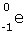, is known as β.This chemical equation represents a potassium-40 atom undergoing beta decay. Notice the β-particle is one product.

To determine the other product, remember that the mass number does not change.

Subtract the atomic number of β from the atomic number of K.

19 – (-1) = 20 is the atomic number of the other product.

To determine the element symbol, look up atomic number 20 on the periodic table.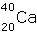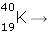Try another one.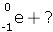Remember that the mass number does not change.

To determine the atomic number of the product, subtract 6 – (-1) = 7.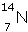To determine the symbol of the product, look up on the periodic table atomic number 7.

An unseen product in beta decay is called a neutrino, and is given the symbol ν. A neutrino is an amount of energy. Remember above, we said that the mass of the parent nuclide changed such a tiny amount that we could ignore that difference when calculating the mass number? How does this fit into the Law of Conservation of Mass? The neutrino is the answer to that. Einstein predicted that mass can sometimes convert into energy. This is what happens during beta decay. That small amount of mass was converted into a neutrino. Sometimes beta decay problems are written showing the neutrino: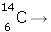## Gamma Decay

Unlike alpha and beta decay, gamma decay does not occur by changing the atomic structure of an atom. A gamma ray, given the symbol γ, is a type of electromagnetic radiation that is emitted when the electric charge of a nucleus is rearranged. Remember that in gamma decay, neither the mass number nor the atomic number are changed.

An equation showing gamma decay might look like this:(source)